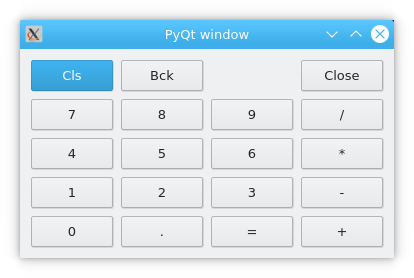# PyQt grid layout (QGridLayout example)

The most frequently used layout class is grid layout, this layout divides the space into rows and columns.

It is an alternative to the box layout and default widget positining.

To create a grid layout, we use the class `QGridLayout`. A grid layout is an evenly divided area to which you can add widgets to each cell.

Book: Create Desktop Apps with Python PyQt5

## QGridLayout example

The example below adds a grid to a PyQt window, it has several rows and columns.We use a for loop to set the grid values, where the contents is in the array names.

``````import sys
from PyQt5.QtWidgets import (QApplication, QWidget,
QPushButton, QGridLayout)

class Example(QWidget):

def __init__(self):
super().__init__()
self.initUI()

def initUI(self):
grid = QGridLayout()
self.setLayout(grid)

names = ['Cls', 'Bck', '', 'Close',
'7', '8', '9', '/',
'4', '5', '6', '*',
'1', '2', '3', '-',
'0', '.', '=', '+',]

positions = [(i, j) for i in range(5) for j in range(4)]

for position, name in zip(positions, names):
if name == '':
continue
button = QPushButton(name)

self.move(300, 150)
self.setWindowTitle('PyQt window')
self.show()

if __name__ == '__main__':
app = QApplication(sys.argv)
ex = Example()
sys.exit(app.exec_())    ``````

In our example, we put the created button controls in a grid.

``````grid = QGridLayout()
self.setLayout(grid)``````

Instantiate QGridLayout and set the layout of the application window.

``````names = ['Cls', 'Bck', '', 'Close',
'7', '8', '9', '/',
'4', '5', '6', '*',
'1', '2', '3', '-',
'0', '.' , '=', '+',]``````

This is the button label to be used later.

``positions = [(i, j) for i in range(5) for j in range(4)]``

We created a list of grid locations.

``````for position, name in zip(positions, names):
if name == '':
continue

button = QPushButton(name)